## Introduction

In classical optics, the polarization state of light is represented by Stokes parameters or the Poincaré sphere1. Pure (linear, circular and elliptical) polarization states correspond to the surface of the sphere while unpolarized light is at the center of the sphere, unbiased relative to any pure polarization state. In quantum optics, polarization of a single photon is expressed by a two-level system, i.e., a qubit2, and the polarization state is represented by a density matrix or a Stokes vector. The unpolarized state of a single photon corresponds to the completely mixed state of a qubit. This means that the unpolarized state of a single photon is a statistical mixture of any two orthogonal polarization bases. If we measure the polarization of a single photon in a certain basis, the measurement outcome will be either of the two orthogonal polarizations with even probability, regardless of the choice of basis. The unpolarized mixed state of a single photon is crucial to explore fundamental problems for mixed states such as testing error-disturbance relations of measurements3,4 and exploring the nature of mixed states themselves5,6, as well as to realize genuine random number generators7,8,9. For these applications, in addition to the statical statistics predicted by the density matrix, we must examine the dynamical statistics of the measurement outcomes. In particular, to ensure true randomness it is essential to show that the polarizations between photons are not correlated with each other. However, to our knowledge, such dynamically unpolarized single photon sources have not been explored to date.

Negatively charged nitrogen-vacancy (NV) centres have received broad attention as single-photon sources over the last few decades10,11,12,13. These properties have allowed NV centres to be used in applications ranging from single-photon sources for quantum cryptography14,15 to tests of the foundations of quantum physics16,17,18. Negatively charged NV centres consist of a substitutional nitrogen atom and an adjacent vacancy with an excess electron in diamond (Fig. 1(a)). When NV centres are non-resonantly excited from the ground state (3A) to the excited state (3E) by a 532 nm laser, the photoluminescence spectrum consists of the zero-phonon line at 637 nm and broad phonon sidebands (600–850 nm) and the lifetime of 3E is 11.6 ns10. The excited states 3E are composed of an orbital doublet of Ex which emits horizontally polarized photons and Ey which emits vertically polarized photons. Electric dipole transitions are allowed for dipoles associated with Ex, Ey in the plane perpendicular to the NV symmetry axis and perpendicular to each other due to the C3v symmetry of the structure of a NV centre19,20 (Fig. 1(a)). In -oriented NV centres, which we used in this study, the orientation of these two dipoles are parallel to the diamond surface21,22,23,24,25.Figure 1: Structure of a -oriented NV centre in diamond and experimental setup.

The degeneracy of the orbital doublet 3E is lifted due to coupling to localized vibrational modes (the Jahn-Teller effect), thereby causing orbital mixing between Ex and Ey within the lifetime of 3E13,24,26,27,28. The polarization visibility of emitted photons from a -oriented NV centre around 4 K is below 60% for both the zero-phonon line under non-resonant excitation24 and the phonon-sidebands under resonant excitation23. This is likely due to the static Jahn-Teller effect13. From around 4 K to 50 K, the polarization visibility decreases to 0% (i.e., unpolarized) exhibiting T5 dependence with thermal equilibration of the orbitals24. This T5 dependence is clear evidence of the dynamic Jahn-Teller effect. This temperature dependence implies that single photons emitted from a -oriented NV centre are also unpolarized at room temperature under non-resonant excitation (Fig. 1(b)). Furthermore, as in thermal noise generators29, it is reasonably expected that truly random selection takes place when photons are emitted from either the Ex or Ey orbital, leading to dynamically unpolarized single photon emission without polarization correlation between time adjacent single photons. However, as mentioned above, polarization correlation between single photons, including those emitted from a -oriented NV centre, has until now never been examined.

## Results and Discussion

### Static polarization measurements

First, we evaluated static polarization properties of emitted photons by measuring polarization angular dependencies, and applying quantum state tomography and quantum process tomography. The excitation by a 532 nm laser and the collection of the phonon sideband emission from single NV centres was carried out by a standard confocal microscopy method at room temperature (See Methods). The detection part of the experimental setup is shown in Fig. 1(c). We used type IIa (111) diamond synthesized by a high pressure high temperature (HPHT) method. In order to search for a -oriented NV centre among four possible 〈111〉 orientations and investigate polarization states of the photons emitted from the NV centre, we measured detection polarization angular dependencies of the fluorescence intensity in both linear and circular polarization bases. In the linear polarization basis measurements, we measured the polarizer angle dependence of the fluorescence intensity by rotating polarizer1 (purple rectangle shown in Fig. 1(c)) for excitation laser polarizations set to horizontal (H), vertical (V), diagonal (D), anti-diagonal (A), right circular (R) and left circular (L). These data are shown in Fig. 2(a). We observed almost no angular dependence, with an average visibility of 6.0% for an excitation power of 1 mW (0.26Psat, where Psat is the saturation excitation power). All measurements conducted in this paper were made for the same -oriented NV centre. Furthermore, we confirmed similar unpolarized properties for several other -oriented NV centres. In the circular polarization basis measurements, we measured the polarizer angle dependence of the fluorescence intensity by rotating polarizer1 followed by a λ/4 waveplate (green rectangle shown in Fig. 1(c)) at 45° for excitation laser polarizations of H, V, D, A, R and L as shown in Fig. 2(b). Again, we found only minimal angular dependence, with an average visibility of 1.3% for an excitation power of 1 mW.

#### Quantum state tomography

We reconstructed a density matrix ρexp for the polarization of the photons emitted from the NV centre from the data shown in Fig. 2(a,b) by a maximum likelihood quantum state tomography method30. The density matrix is shown in Fig. 2(c). The density matrix shows that the polarization state is nearly the completely mixed state which is given by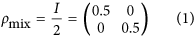in either the R, L basis or the H, V basis, where I is the two-dimensional identity matrix. The Stokes vector calculated from the density matrix is (S1/S0, S2/S0, S3/S0) = (0.057, −0.003, −0.004). This vector is located near to the origin of the Poincaré sphere, indicating an almost completely unpolarized state. We also estimated the average fidelity. Therefore, the single photons emitted from the measured single -oriented NV centre are statistically statically unpolarized.

#### Quantum process tomography

Next, in order to find a key quantum operator describing the excitation-emission process of the NV centre, we evaluated the χ matrix by quantum process tomography31,32 (See Methods). The input state of the process is assumed to be the polarization state of the 532 nm excitation laser and the output state of the process is assumed to be single photons emitted from the NV centre. The ideal χ matrix χideal for the process, which outputs the completely mixed state ρmixed out for any input ρin, is diagonal with all entries equal to 1/4 because of the identity relation ρmixed out = (inI + inX + inY + inZ)/4 = I/2, where X, Y and Z are the Pauli matrices, which form the basis of the χ matrix. χexp, which is the χ matrix estimated from the data shown in Fig. 2(a,b), is shown in Fig. 2(d). The result demonstrates that the process outputs a nearly completely mixed polarization state of emitted single photons for any input excitation polarization state. We also estimated the process fidelity33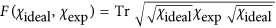= 0.999.

### Dynamical polarization correlation measurements

Finally, in order to demonstrate the dynamical nature of the randomness of the emitted photon polarization, we measured the polarization correlation between time adjacent emitted single photons by measuring the modified second-order correlation function g(2)(τ) using the setup shown in Fig. 1(c). The basic setup was a standard Hanbury Brown-Twiss type setup in which the photon stream was divided by the insertion of a non-polarizing beamsplitter (NPBS), and detected at the outputs by avalanche photodiodes (APD1 and APD2). For the polarization correlation measurements, polarizer2, 3 (thick blue rectangles shown in Fig. 1(c)) were introduced between the NPBS and the APDs. We measured the modified second-order correlation function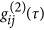at weak excitation (0.20 mW = 0.05Psat), where ij denotes the polarizer settings HH, VV, HV and VH and the first (second) character denotes the orientation of polarizer2 (polarizer3). This means that if the polarizer settings are HV, APD1 detects only H polarization photons and APD2 detects only V polarization photons and g(2)(τ) is measured for these photons. If there is polarization correlation, for example time adjacent photons tend to have same H polarization, HH, the temporal profile of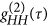would give a narrower anti-bunching dip (i.e. a smaller decay time) than that of others,and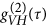. Thereby we can investigate dynamic polarization correlation using this method. Thedata we measured are shown in Fig. 3(a). The data show that the fourare identical to each other within experimental accuracy. The average of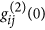over the four polarizer settings was found to be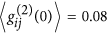. Note that the measured value of g(2)(0) < 0.5 (anti-bunching) indicates strong evidence of single photons for all polarizer settings. These data indicate that time adjacent emitted single photons have essentially no polarization correlation. We also measured under strong excitation conditions (8.8 mW = 2.3Psat) as shown in Fig. 3(b). In addition to the anti-bunching dip with, bunching occurred around the anti-bunching dip due to optical cycling in the three-level system10. Again, we found that the fourwere identical to each other within experimental accuracy.

Usual g(2)(τ) data for emitted singe photons from a single NV centre are fitted by the following function which is derived by analysis of the three-level system,where τa is the decay time of anti-bunching, τb is the decay time of bunching, c is the coefficient of bunching and ρ is signal-to-background ratio10,34. For our cases, thedata were fitted by Eq. (2). Decay times τa of anti-bunching for the four polarizer settings (estimated by fitting for thedata shown in Fig. 3(a,b)) are shown in Fig. 3(c,d), respectively. These figures show that the decay times for the four polarizer settings are equal within the estimated standard error of the fitted values. These results are consistent with the expectation of uncorrelated decay times and we therefore conclude that the emitted single photons are dynamically unpolarized for weak and strong excitation cases.

Next, we evaluated the polarization correlation between same and different polarizations using a polarization correlation function C(τ) defined by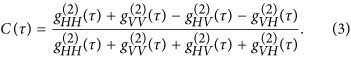C(τ) is a quantitative measure of the dynamical polarization correlation. In particular, if measured photons have exactly the same (different) polarization, i.e., HH or VV (HV or VH), C(τ) is equal to +1 (−1) for any τ. On the other hand, if the measured photons have no polarization, C(τ) is equal to 0. C(τ) values for our data under weak and strong excitation condition are shown in Fig. 3(e,f), respectively. The data show that C(τ) is close to 0 for all τ that we measured; C(τ) averaged over all τ is 0.0008, 0.0022 and the standard deviation is 0.0206, 0.0109 for weak and strong excitation, respectively. Almost all of the 90% confidence intervals for C(τ) values (shaded area in Fig. 3(e,f)) include 0. Note that the slight peak and dip seen around τ = 0 in Fig. 3(e,f) respectively are due to fluctuations in the background which become prominent due to the vanishing correlation signal at τ = 0. We have therefore demonstrated essentially no polarization correlation between time adjacent emitted single photons for both weak and strong excitation conditions.

## Conclusion

We have presented the first demonstration of unpolarized single photons emitted from a -oriented NV centre in diamond in both a statical and dynamical sense. The new ‘dynamically unpolarized’ property, i.e. the property of no polarization correlation between time adjacent single photons, constitutes a new criterion of randomness for unpolarized single photons which is useful for true random number generation7,8,9 as well as for tests of fundamental quantum mechanics pertaining to mixed states3,4,5,6.

## Methods

### Confocal microscopy

A continuous-wave 532 nm laser was used for non-resonant excitation of the NV centre. The laser was focused with a 1.4 numerical aperture (NA) oil immersion objective lens, which also collected the photons emitted from a single NV centre. Using band-pass filters, photons emitted from the NV centre ranging from 650 nm to 800 nm were measured. The measurements of the second-order correlation functionfor the filtered photons were performed using a modified Hanbury Brown-Twiss type setup as explained in the main text.

### Quantum process tomography

Quantum process tomography is a method for estimating the χ matrix, which finds quantum operators describing a given quantum process31,32. The processfor the one qubit case, having input state ρin and output state ρout can be written as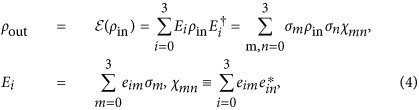where σm,n {I, X, Y, Z}. Here, I is the two-dimensional identity matrix and X, Y and Z are the Pauli matrices, which form the basis of the χ matrix.

For our case, accurately speaking, the polarization state of this laser is a classical polarization rather than a quantum state. However the excitation-emission process is a linear process which means that a single-photon in the excitation laser is absorbed by a NV center and subsequently a single-photon is emitted from the NV center. In this case the polarization state of the absorbed single photon is identical to that of the excitation laser. This allows us to evaluate correlations arising from the process of excitation followed by emission of a single photon. We followed the concrete procedure of quantum process tomography given in ref. 32.